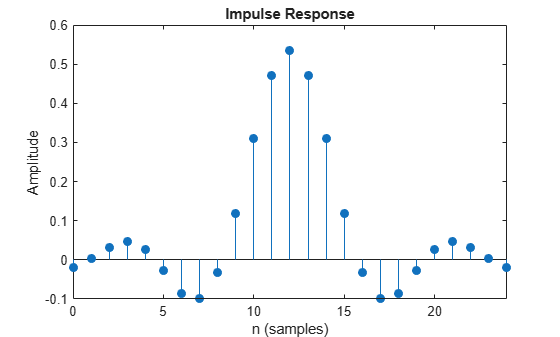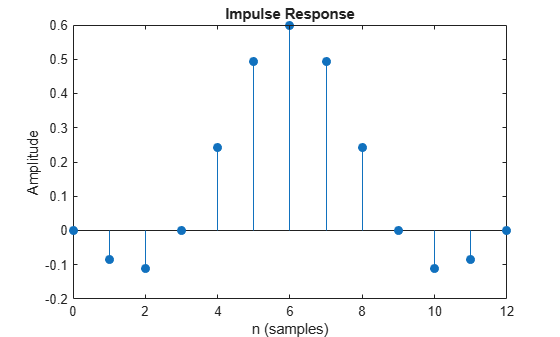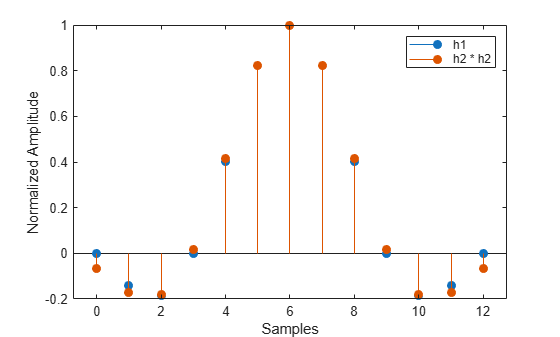# rcosdesign

Raised cosine FIR pulse-shaping filter design

## Syntax

``b = rcosdesign(beta,span,sps)``
``b = rcosdesign(beta,span,sps,shape)``

## Description

example

````b = rcosdesign(beta,span,sps)` returns the coefficients, `b`, that correspond to a square-root raised cosine FIR filter with rolloff factor specified by `beta`. The filter is truncated to `span` symbols, and each symbol period contains `sps` samples. The order of the filter, `sps*span`, must be even. The filter energy is 1.```

example

````b = rcosdesign(beta,span,sps,shape)` returns a square-root raised cosine filter when you set `shape` to `'sqrt'` and a normal raised cosine FIR filter when you set `shape` to `'normal'`.```

## Examples

collapse all

Specify a rolloff factor of 0.25. Truncate the filter to 6 symbols and represent each symbol with 4 samples. Verify that `'sqrt'` is the default value of the `shape` parameter.

```h = rcosdesign(0.25,6,4); mx = max(abs(h-rcosdesign(0.25,6,4,'sqrt')))```
```mx = 0 ```
`fvtool(h,'Analysis','impulse')`Compare a normal raised cosine filter with a square-root cosine filter. An ideal (infinite-length) normal raised cosine pulse-shaping filter is equivalent to two ideal square-root raised cosine filters in cascade. Thus, the impulse response of an FIR normal filter should resemble that of a square-root filter convolved with itself.

Create a normal raised cosine filter with rolloff 0.25. Specify that this filter span 4 symbols with 3 samples per symbol.

```rf = 0.25; span = 4; sps = 3; h1 = rcosdesign(rf,span,sps,'normal'); fvtool(h1,'impulse')```The normal filter has zero crossings at integer multiples of `sps`. It thus satisfies Nyquist's criterion for zero intersymbol interference. The square-root filter, however, does not:

```h2 = rcosdesign(rf,span,sps,'sqrt'); fvtool(h2,'impulse')```Convolve the square-root filter with itself. Truncate the impulse response outward from the maximum so it has the same length as `h1`. Normalize the response using the maximum. Then, compare the convolved square-root filter to the normal filter.

```h3 = conv(h2,h2); p2 = ceil(length(h3)/2); m2 = ceil(p2-length(h1)/2); M2 = floor(p2+length(h1)/2); ct = h3(m2:M2); stem([h1/max(abs(h1));ct/max(abs(ct))]','filled') xlabel('Samples') ylabel('Normalized amplitude') legend('h1','h2 * h2')```The convolved response does not coincide with the normal filter because of its finite length. Increase `span` to obtain closer agreement between the responses and better compliance with the Nyquist criterion.

This example shows how to pass a signal through a square-root, raised cosine filter.

Specify the filter parameters.

```rolloff = 0.25; % Rolloff factor span = 6; % Filter span in symbols sps = 4; % Samples per symbol```

Generate the square-root, raised cosine filter coefficients.

`b = rcosdesign(rolloff, span, sps);`

Create a vector of bipolar data.

`d = 2*randi([0 1], 100, 1) - 1;`

Upsample and filter the data for pulse shaping.

`x = upfirdn(d, b, sps);`

`r = x + randn(size(x))*0.01;`

Filter and downsample the received signal for matched filtering.

`y = upfirdn(r, b, 1, sps);`

For information on how to use square-root, raised cosine filters to interpolate and decimate signals, see Interpolate and Decimate Using RRC Filter (Communications Toolbox).

## Input Arguments

collapse all

Rolloff factor, specified as a real nonnegative scalar not greater than 1. The rolloff factor determines the excess bandwidth of the filter. Zero rolloff corresponds to a brick-wall filter and unit rolloff to a pure raised cosine.

Data Types: `double` | `single`

Number of symbols, specified as a positive integer scalar.

Data Types: `double` | `single`

Number of samples per symbol (oversampling factor), specified as a positive integer scalar.

Data Types: `double` | `single`

Shape of the raised cosine window, specified as either `'normal'` or `'sqrt'`.

## Output Arguments

collapse all

Raised cosine filter coefficients, returned as a row vector.

Data Types: `double` | `single`

## Tips

• If you have a license for Communications Toolbox™ software, you can perform multirate raised cosine filtering with streaming behavior. To do so, use the System object™ filters, `comm.RaisedCosineTransmitFilter` and `comm.RaisedCosineReceiveFilter`.

 Tranter, William H., K. Sam Shanmugan, Theodore S. Rappaport, and Kurt L. Kosbar. Principles of Communication Systems Simulation with Wireless Applications. Upper Saddle River, NJ: Prentice Hall, 2004.